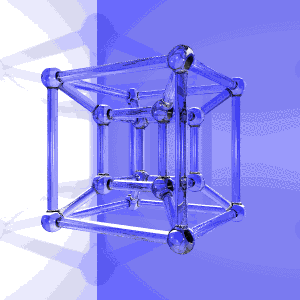# ALESSANDRUINO INVERSE KINEMATIC SUPA-FLY :D

UPDATE 06.19.13

GET RID OF YOUR 2 DOF INVERSEKINEMATICS ENGINE !!!!

NOW WE HAVE THE 10 DOF ENGINE :D X,Y AND ANGLE OF EACH JOINT :D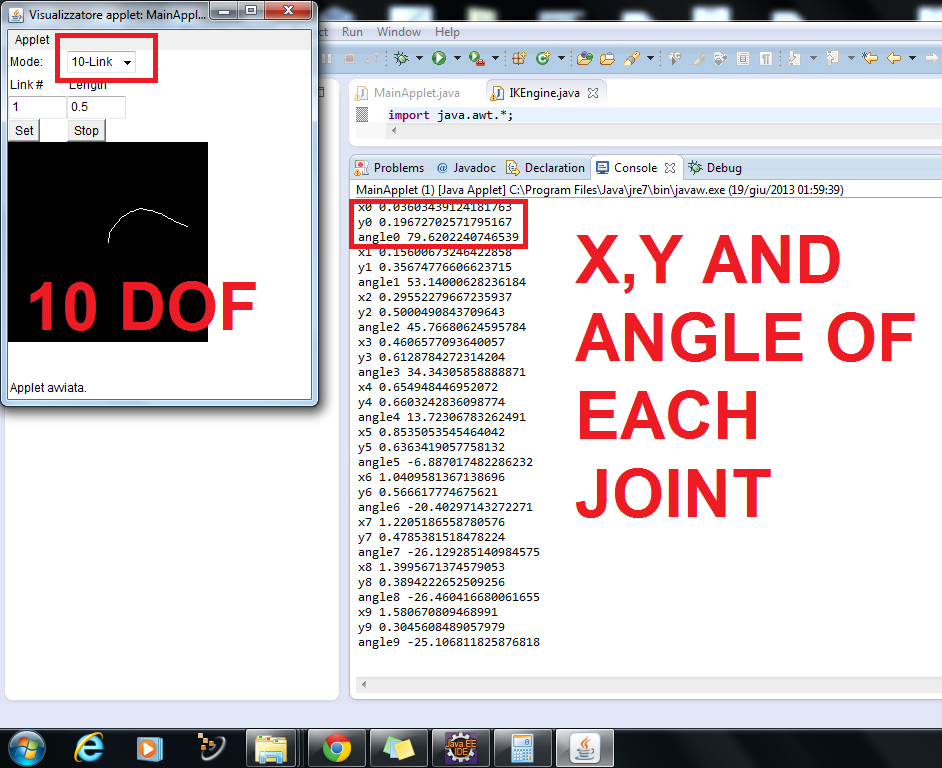UPDATE 06.15.2013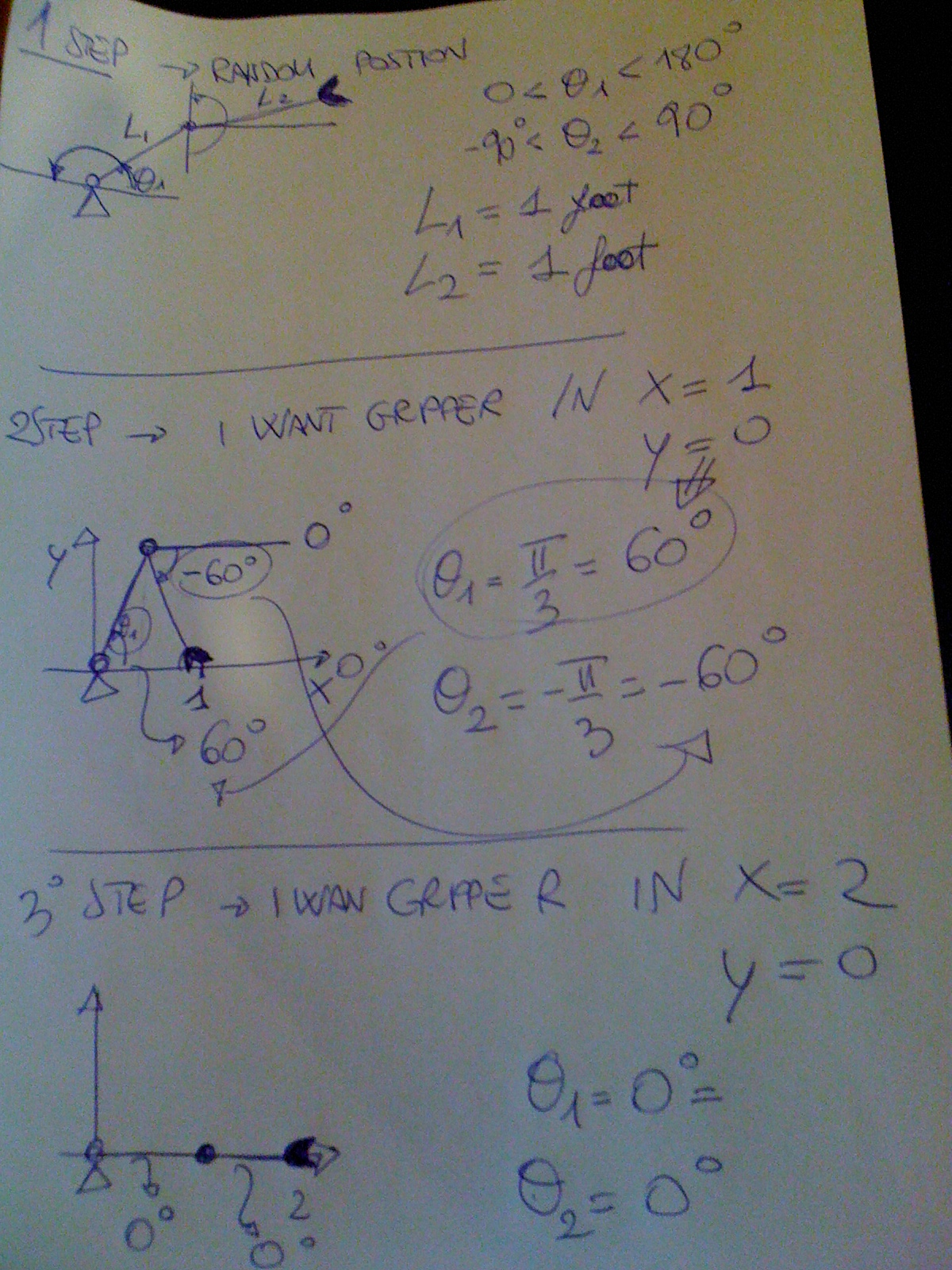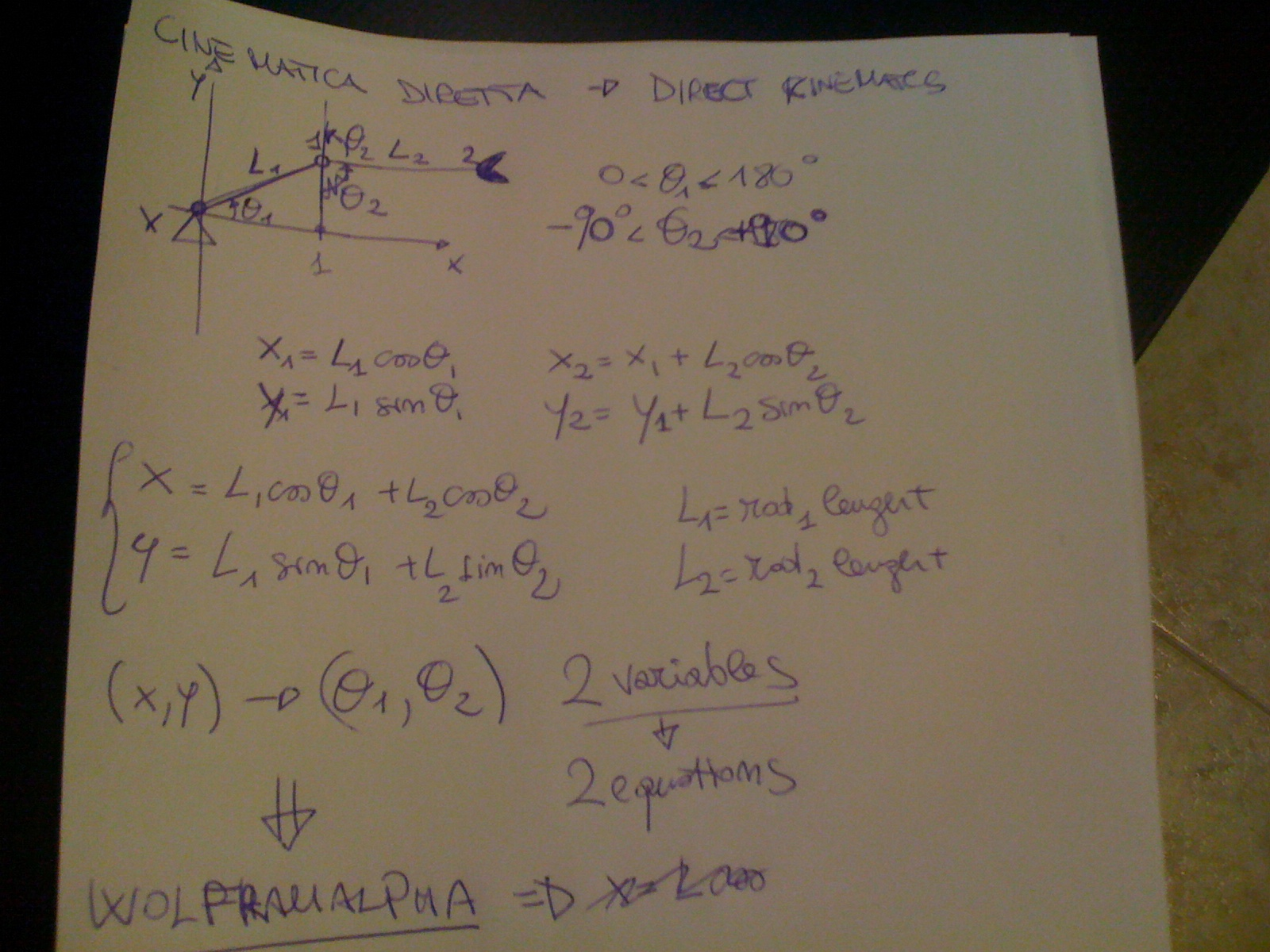UPDATE 06.15.2013

Today i decided to get rid of the javafx-inversekinematics library-because it is more for graphic use than for calculus.

I'll try with jroboop library later...for the moment i'm back with the "HARDWARE" mode...

I wrote the kinematics equations for 2 rods with 2 joints...

L1 AND L2 are the 2 rods lenght

Teta1 and teta2 are 2 angles

the 2 disequations set the min and the max for the angles...

Once setted a (X,Y) Point , Then i'll let wolframpalpha service calculate the inverse equation to find angles of the rods to reach that point

In the example at the bottom i give lenght of the rods (lenght = 1), and point X=1, Y=0

The angles from inverse kinematics are teta1 = 60 degreeses( pi/3) and teta2 = -60 degreeses (-pi/3)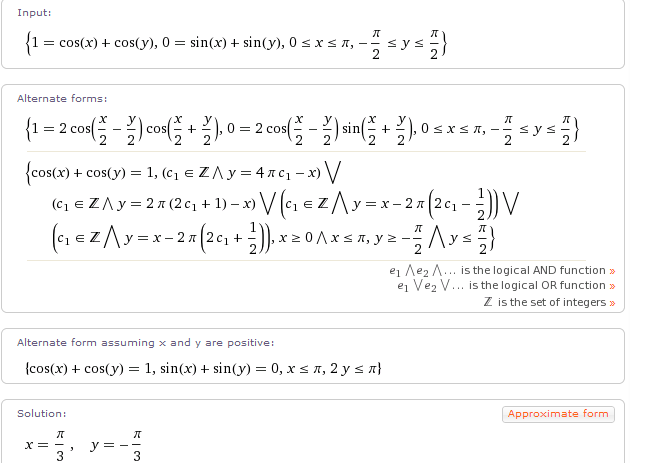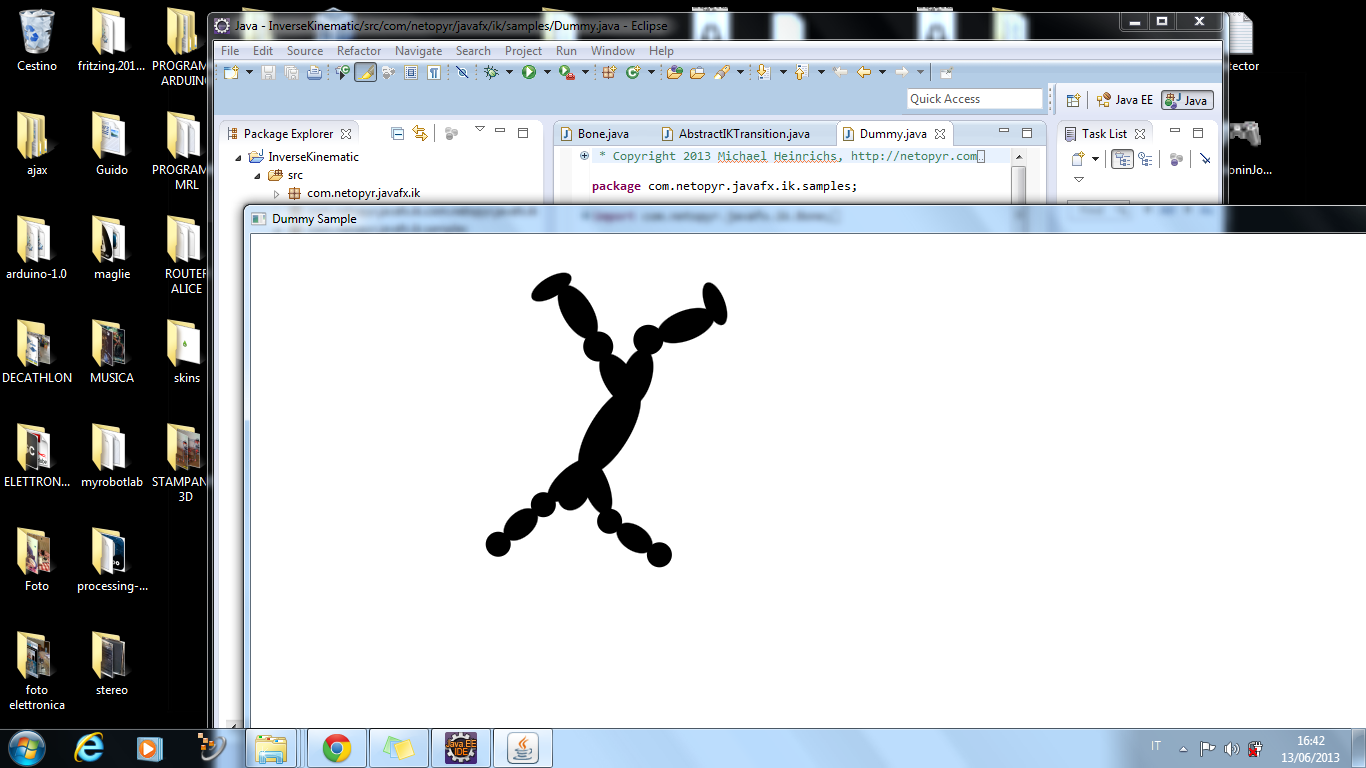)

## Comment viewing options